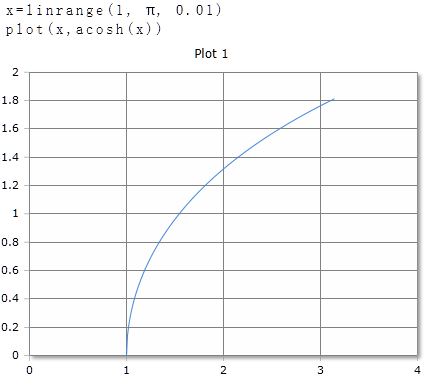# ACosh Function

Computes the inverse hyperbolic cosine

## Description

The function $$ACosh$$ calculates the angle to the hyperbolic cosine of the argument.

$$ACosh$$ returns the angle to the hyperbolic cosine of real or complex numbers. The argument can be a single number or a data field. For data fields, the inverse hyperbolic cosine of each element is calculated and the results are returned in a data field of equal size.

## ACosh for real numbers

### Parameter

The argument must be greater than or equal to 1. If the value is less than 1, the function returns the result NaN (not a number).

### Result

The result is given in degrees (full circle = 360 °) or radians / radians (full circle = 2 · π). The unit of measure used is set in the toolbar with the DEG or RAD buttons or an optional parameter. The setting in the toolbar applies to the entire worksheet.

### Optional Parameter

Optionally, a second parameter can be specified with the keywords DEG or RAD to set the unit of measure for this function call. The specification of the parameter has priority over the global setting in the toolbar. You can use it on a worksheet different functions use different units of measurement independent of the presetting in the toolbar.

### Syntax

ACosh (value)

ACosh (value, DEG)

### Example## ACosh for complex numbers

For complex numbers, the result is always given as radians, regardless of the default setting in the toolbar. The result is also a complex number.

ACosh (re + im)

### Example

ACosh(3 + 4i)= 2.31+0.937i